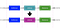# What’s Functional Programming?

## Isolate and replaceEasy to isolate errors and replace the defective component — Image by the author

## Reducing complexity

`f(x) = (2x+3)g(x) = x²h(x) = (g º f)(x) =  g(f(x)) = (2x+3)²`
`const finalOutput = double º sort º display = display(sort(double(array)))`

# Higher-Order Functions

“Functions that operate on other functions, either by taking them as arguments or by returning them, are called higher-order functions.” — Eloquent JavaScript

`const data = [1, 4, 2, 8];const double = x => x * 2;const plusOne = x => x + 1;console.log('double array : ', transform(data, double));console.log('plus one array : ', transform(data, plusOne));`

## Higher-order functions in JavaScript

`const sequence = [1, 2, 3, 4, 5];const doubleSequence = sequence.map((item) => item * 2); // [2,4,6,8,10]`
`const isString = value => typeof value === 'string';const values = [12, 'Hi', 1, 'Sun', 'Sky', 8];const valuesMatched = values.filter(isString);console.log('valuesMatched : ', valuesMatched); // "valuesMatched : ", ["Hi", "Sun", "Sky"]`
`[12, 5, 8, 130, 44].every(elem => elem >= 10); // false[12, 54, 18, 130, 44].every(elem => elem >= 10); // true[{a:1, b:2}, {a:1, b:3}].every(elem => elem.a === 1); // true[{a:2, b:2}, {a:1, b:3}].every(elem => elem.a === 1); // false`
`[2, 5, 8, 1, 4].some(elem => elem > 10); // false[12, 5, 8, 1, 4].some(elem => elem > 10); // true (12)`

# How to Chain Functions

`const complexArray = [6, 2, 4, 8].map(x => x * 2).map(x => x + 1).filter(x => x > 10);console.log('complexArray : ', complexArray); // "complexArray : ", [13, 17]`

# Safety in Functional Programming

## Functors

`const increment = v => v + 1increment('5') // '51'increment({ v: 5 }) // "[object Object]1"`
`const double = (v) => {  if (typeof v !== 'number') {    return NaN  }  return v * 2}`
`const safeResult = NumberBox({  v: 5}).map(v => v * 2) // -> executed.map(v => v + 1) // -> executed.value // NaN`

`MayBe(Backend.Call()).map().map().getOrElse();`

# Conclusion

## Better Programming

### By Better Programming

A weekly newsletter sent every Friday with the best articles we published that week. Code tutorials, advice, career opportunities, and more! Take a look

Written by

Written by

## Héla Ben Khalfallah

#### I love coding whatever the language and trying new programming tendencies. I have a special love to JS (ES6+), functional programming, clean code & tech-books.